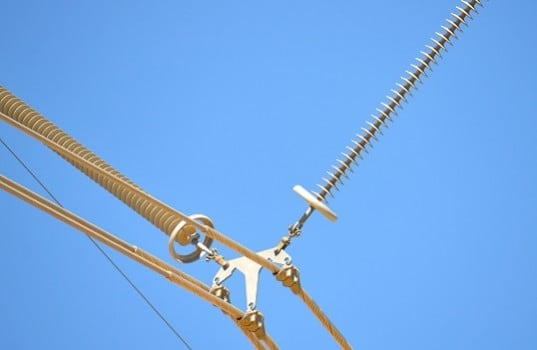## Electric Field & Voltage Distribution Along Non-Ceramic Insulators

July 15, 2017 • ARTICLE ARCHIVE, InsulatorsThe equipotential contours around the five computation models are shown in Fig. 4. Again, the bottom electrode is energized, the top electrode grounded. The energizing voltage is 1000 V and the insulation distance between the two electrodes is 46 cm. Each number shown along the perimeters of the four contour plots is in centimeters. Case (a), no solid insulating material between the electrodes, shows that only some 20 per cent of the insulation distance sustains about 70 per cent of the applied voltage. The presence of the fiberglass rod changes the voltage distribution slightly, see Case (b).

The distribution of the equipotential contours for Case (c), with the sheath on the rod, is very close to Case (e), the “full” insulator model. The presence of the weather sheds changes the equipotential contours somewhat. If more accurate results of voltage distribution are needed near the line and ground end areas, the simplified insulator model with only two weather sheds at each end of the insulator, Case (d), can be used. Comparing Cases (d) and (e), the voltage distributions in the vicinity of the two weather sheds at the line end are very similar. Moreover, the positions of the equipotential lines for Cases (d) and (e) are very close to one another along the surface of the insulator’s sheath.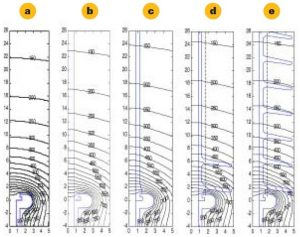Fig. 4: The equipotential contours around the five computation models.
CLICK TO ENLARGE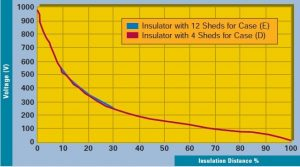Fig. 5: Voltage magnitude along insulation distance at surface of sheath for Cases (d) and (e).
CLICK TO ENLARGE

To see the comparison more clearly, the voltage distributions for Cases (d) and (e) along the calculation paths, shown as a dashed line in Fig. 4 (d) and (e), are shown in Fig. 5. Comparing Cases (d) and (e), the maximum difference between the voltages at the same point along the sheath surface of the insulator is only 1.2 per cent of the applied voltage. This indicates that the simplification introduced by Case (d) is acceptable for the calculation of the voltage distribution of the “full” insulator, Case (e), along the sheath surface.

The electric field strength magnitudes for Cases (d) and (e) along the paths defined on the surface of the sheath are also calculated for comparison, (see Figure 6.) The dips in the electric field strength plot of the insulator modeled with weather sheds are due to the calculation path passing through the weather shed material, which has a relative permittivity of 4.3. The electric field strength values in the vicinity of the two weather sheds at each end of the insulator are the same for Cases (d) and (e). There is a slight change in the electric field strength distribution near the other eight weather sheds shown by Case (e). The maximum electric field strength for Cases (d) and (e) are both the same, i.e.0.0256 kVp/mm. This means that the electric field distribution of the insulator with the “full” number of weather sheds can be estimated through the simplified insulator model with a smaller number of weather sheds (e.g. only two) at each end of the insulator.

The conclusion which can be reached is that a simplified insulator model with only a small number of weather sheds (shown in Fig. 2d), can be used to calculate the electric field and voltage distribution along the “full” insulator in service, with no significant influence on accuracy. The number of weather sheds for the simplified insulator model can be decided simply by trial and error.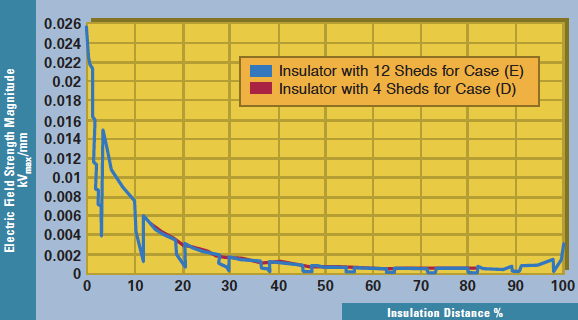Fig. 6: Electric field strength magnitude along the insulation distance at the sheath surface for the “full” insulator, Case (e), and the simplified insulator model, Case (d).
CLICK TO ENLARGE

## Computation Model of 765 kV Composite Insulator

The following discussion describes research related to determining the EFVD along typical 765 kV composite insulators when installed on a three-phase energized tower. The effects of tower configuration and other line components on EFVD are also analyzed. It is of practical interest to know the electric field strength distribution for a full-scale insulator under three-phase energization. A typical 765 kV composite insulator is used for this study, which is designed for four-sub-conductor bundles.

The detailed dimensions of the 765 kV insulator are shown in Fig. 7. Materials used are silicone rubber weather sheds and sheath, and FRP rod. There are over 100 weather sheds (large and small) on an actual 765 kV insulator. Based on the previous analysis, the calculation model for this full scale insulator can be simplified with only a small number of weather sheds (e.g. 10) at each end of the insulator in order to calculate the EFVD.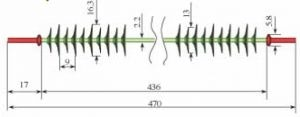Fig. 7: Simplified geometry and dimensions of 765 kV composite insulator model with 10 weather sheds at line and ground ends.
CLICK TO ENLARGE

In order to reduce the electric field strength in the triple point region of the insulator, corona rings are applied at both line and ground ends of the insulator.

1

2

3